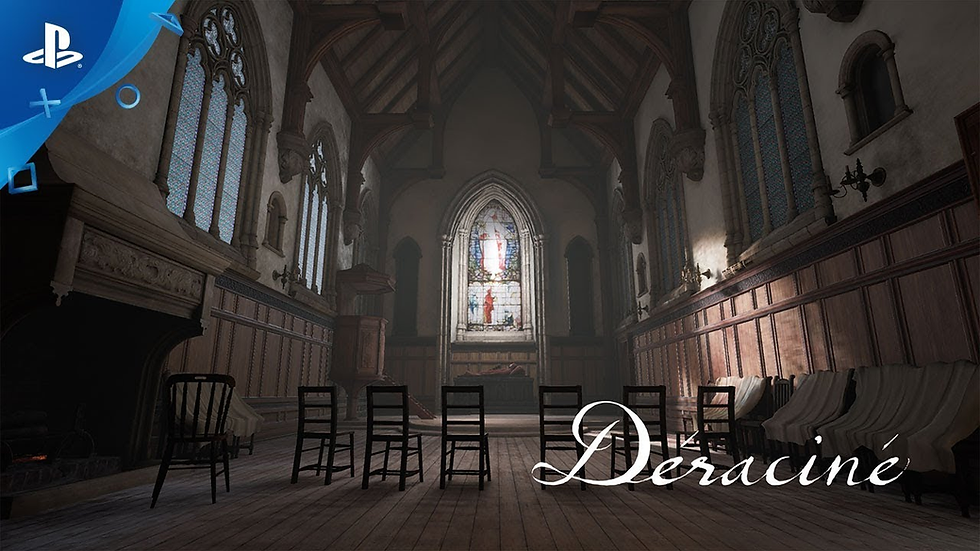top of page

# 宮崎英高Miyazaki Hidetaka製作遊戲合集## 以下為宫崎老師的著名代表作

2009 惡魔靈魂 | Demon's Souls==|====|====|====|====|====|====|====|====|====|====|====|====|====|===

2011 黑暗靈魂 | Dark Souls==|====|====|====|====|====|====|====|====|====|====|====|====|====|===

2014 黑暗靈魂II | Dark Souls II==|====|====|====|====|====|====|====|====|====|====|====|====|====|===

2015 血源詛咒 | Bloodborne==|====|====|====|====|====|====|====|====|====|====|====|====|====|===

2016 黑暗靈魂III | Dark Souls III==|====|====|====|====|====|====|====|====|====|====|====|====|====|===

2018 Déraciné==|====|====|====|====|====|====|====|====|====|====|====|====|====|===

2019 隻狼：暗影雙死 | Sekiro Shadows Die Twice

20年後，由於一心的衰老和染病以及內府勢力的圍剿，葦名國處於崩壞的邊緣。為重振葦名國，葦名一心之孫葦名弦一郎綁架了卿子九郎，希望利用他體內能使人獲得不死之力的「龍胤」來製造不死的軍隊。此時，狼已成長為成熟的忍者，潛進葦名城營救九郎但在城外被弦一郎阻止，不但落敗而且被砍去左臂。由於狼三年前已獲九郎授予了龍胤，因此活了下來並在一古廟甦醒，發現斷臂已被裝上義肢；廟中不斷雕刻著佛像的獨臂佛雕師本為忍者，在發現命危的狼後把他救回並贈予其忍義手。。。。。。==|====|====|====|====|====|====|====|====|====|====|====|====|====|===

2022 艾爾登法環 | Elden Ringbottom of page